# Object Oriented Programming Lecture 2 Object Oriented Design

• Slides: 54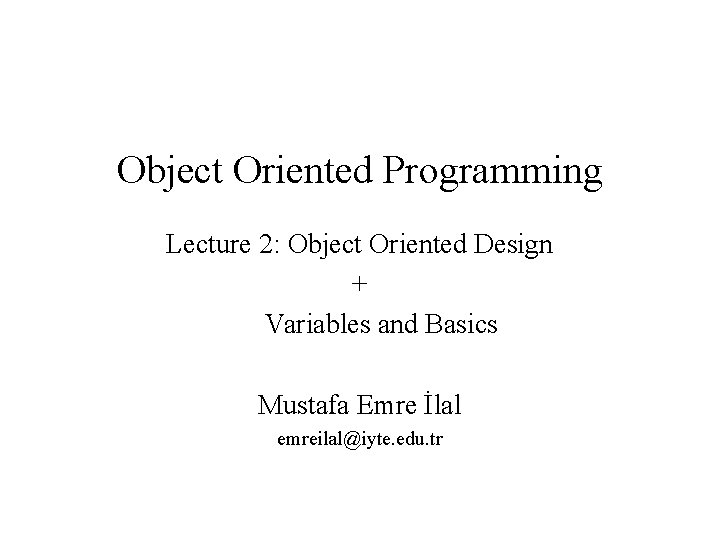Object Oriented Programming Lecture 2: Object Oriented Design + Variables and Basics Mustafa Emre İlal [email protected] edu. tr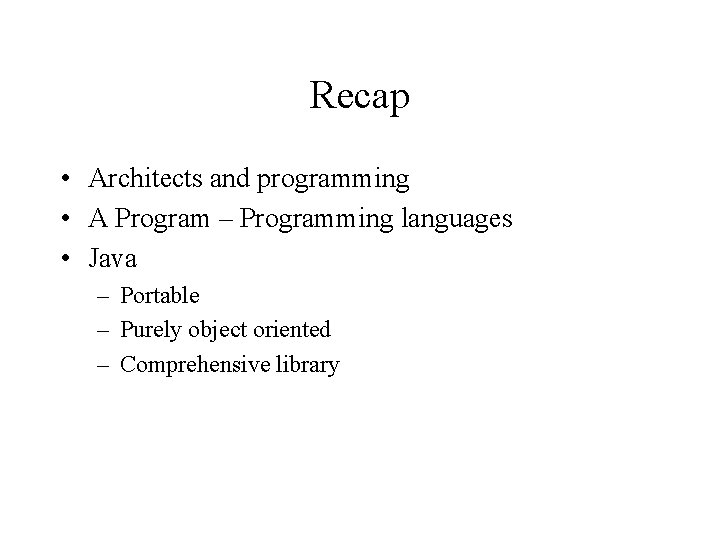Recap • Architects and programming • A Program – Programming languages • Java – Portable – Purely object oriented – Comprehensive libraryToday • 1. Half: Object Oriented Design – Software Engineering • 2. Half: Variables and basics – Your first program in Java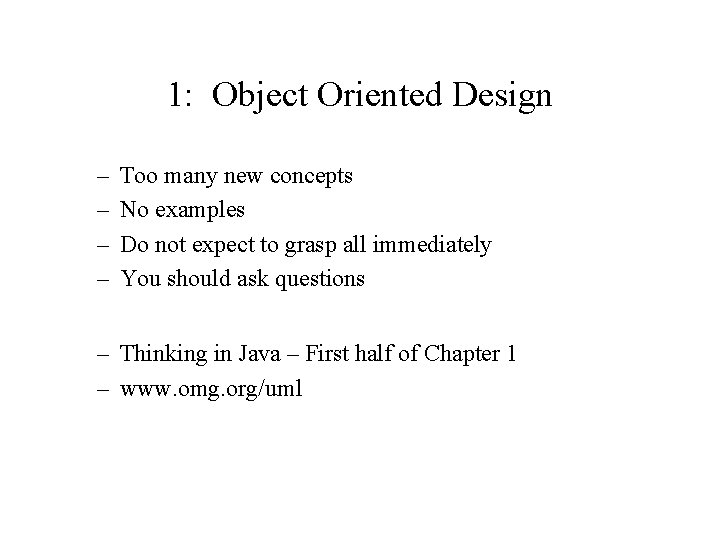1: Object Oriented Design – – Too many new concepts No examples Do not expect to grasp all immediately You should ask questions – Thinking in Java – First half of Chapter 1 – www. omg. org/umlProgramming • Problem solving • Architecture = Programming? • Constrained by mathematical models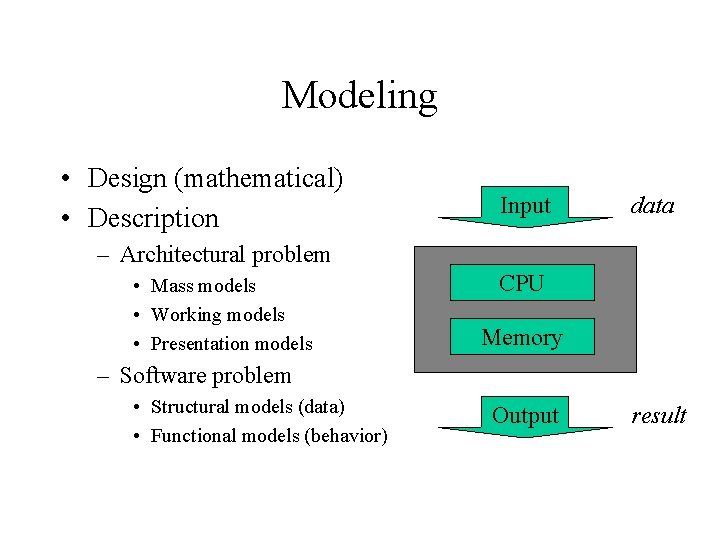Modeling • Design (mathematical) • Description Input data – Architectural problem • Mass models • Working models • Presentation models CPU Memory – Software problem • Structural models (data) • Functional models (behavior) Output resultUML • Unified Modeling Language • Booch, Rumbaugh and Jacobson • Common “language” for describing object oriented systems • Representation is mostly through visual diagrams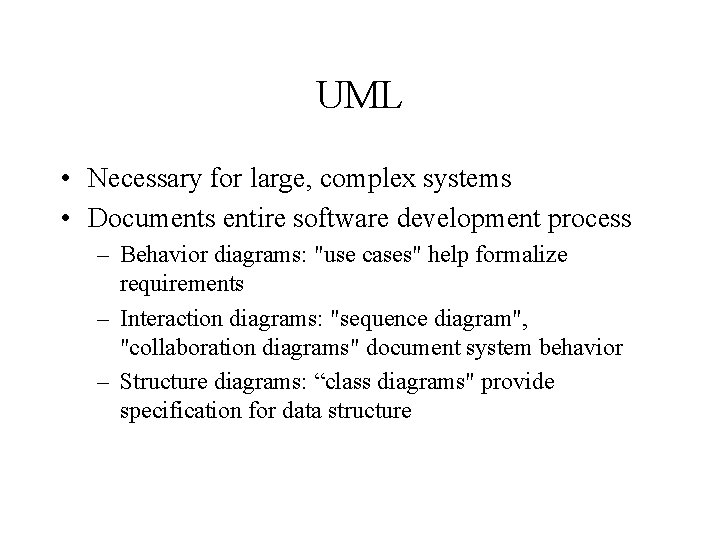UML • Necessary for large, complex systems • Documents entire software development process – Behavior diagrams: "use cases" help formalize requirements – Interaction diagrams: "sequence diagram", "collaboration diagrams" document system behavior – Structure diagrams: “class diagrams" provide specification for data structure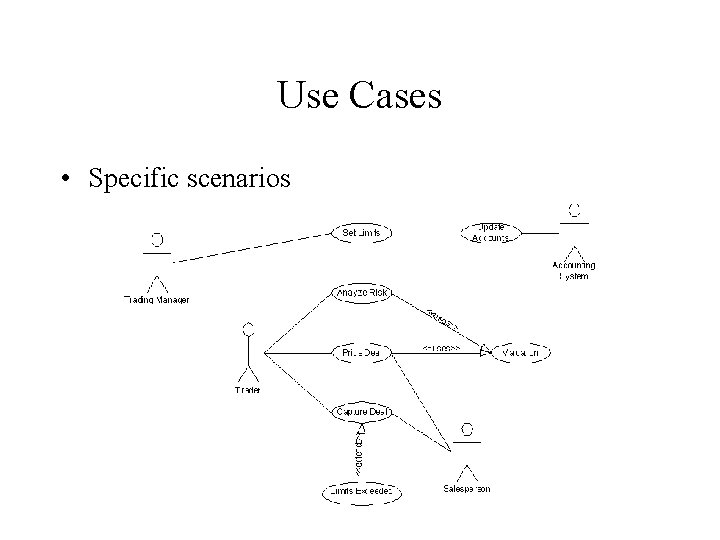Use Cases • Specific scenarios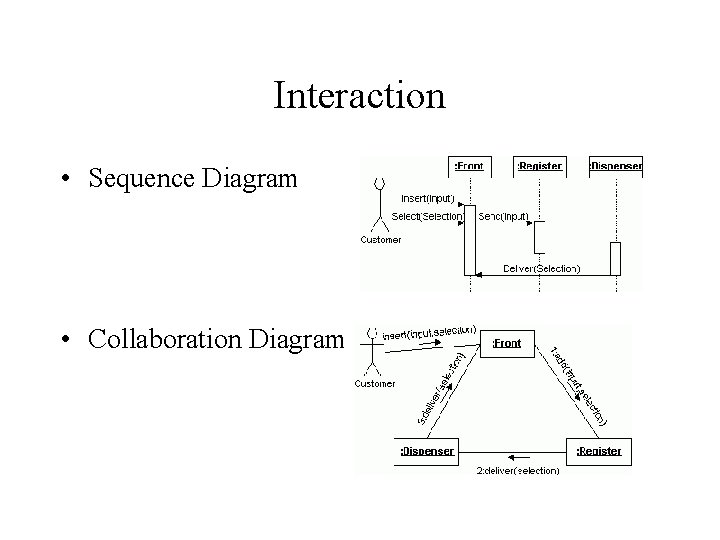Interaction • Sequence Diagram • Collaboration DiagramClass Diagrams • Data Structures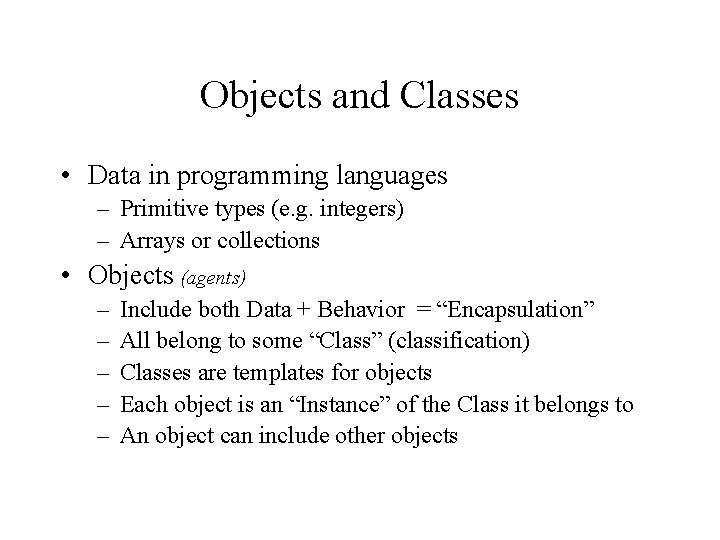Objects and Classes • Data in programming languages – Primitive types (e. g. integers) – Arrays or collections • Objects (agents) – – – Include both Data + Behavior = “Encapsulation” All belong to some “Class” (classification) Classes are templates for objects Each object is an “Instance” of the Class it belongs to An object can include other objects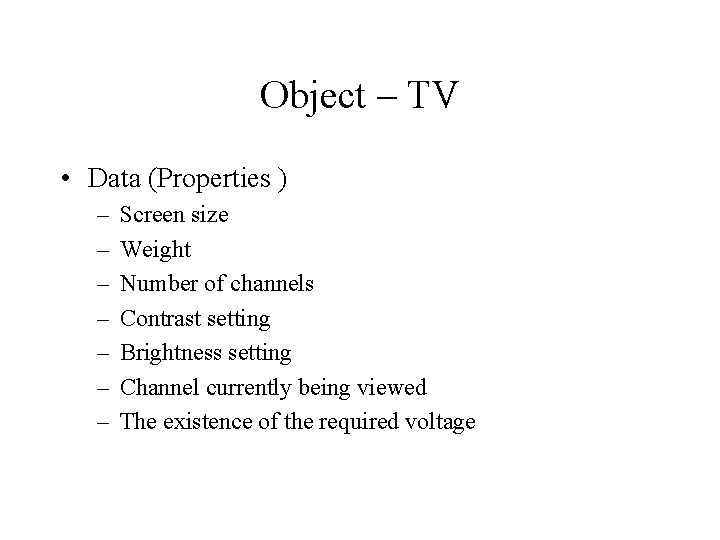Object – TV • Data (Properties ) – – – – Screen size Weight Number of channels Contrast setting Brightness setting Channel currently being viewed The existence of the required voltage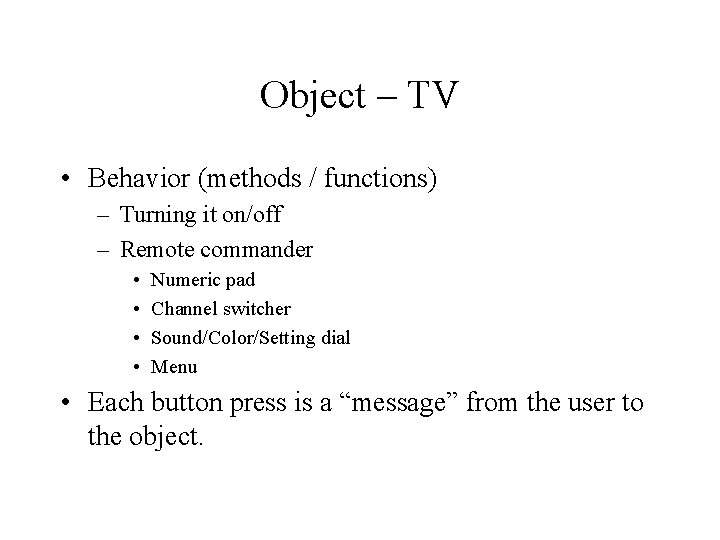Object – TV • Behavior (methods / functions) – Turning it on/off – Remote commander • • Numeric pad Channel switcher Sound/Color/Setting dial Menu • Each button press is a “message” from the user to the object.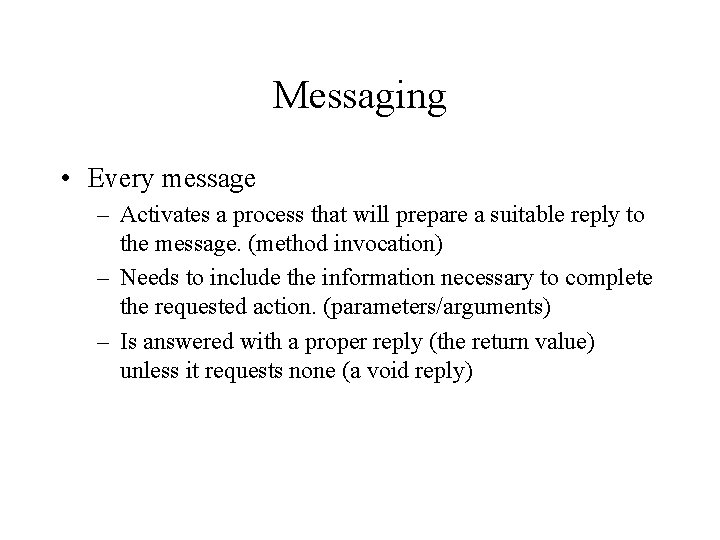Messaging • Every message – Activates a process that will prepare a suitable reply to the message. (method invocation) – Needs to include the information necessary to complete the requested action. (parameters/arguments) – Is answered with a proper reply (the return value) unless it requests none (a void reply)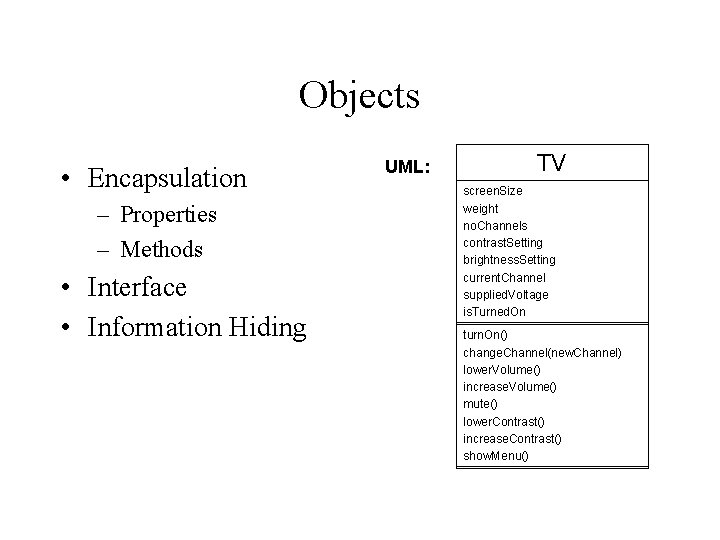Objects • Encapsulation – Properties – Methods • Interface • Information Hiding UML: TV screen. Size weight no. Channels contrast. Setting brightness. Setting current. Channel supplied. Voltage is. Turned. On turn. On() change. Channel(new. Channel) lower. Volume() increase. Volume() mute() lower. Contrast() increase. Contrast() show. Menu()Information Hiding • The object interface is a “contract” between the developer of the class and the other developers • There needs to be a way to be able to make changes to the class without breaking contract. • Java keywords for access: – – public protected private “default" or “friendly”– when nothing is specified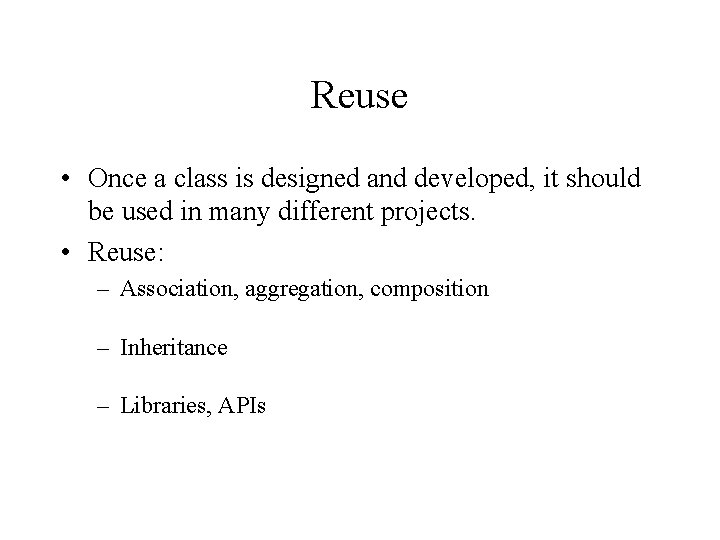Reuse • Once a class is designed and developed, it should be used in many different projects. • Reuse: – Association, aggregation, composition – Inheritance – Libraries, APIs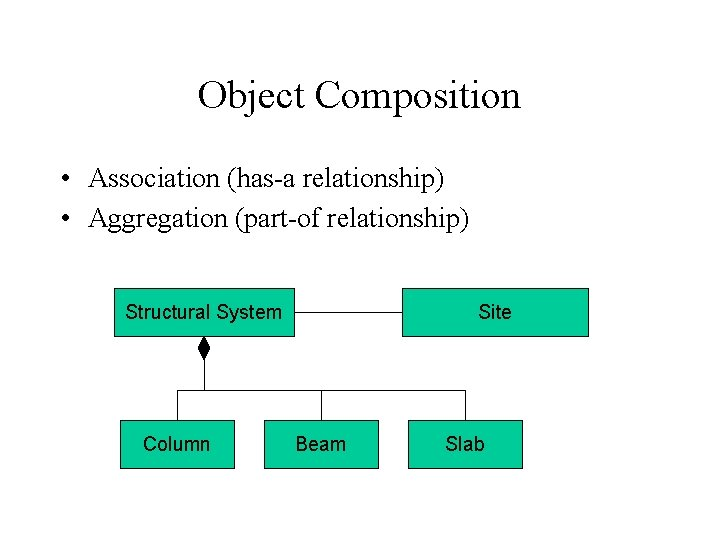Object Composition • Association (has-a relationship) • Aggregation (part-of relationship) Structural System Column Site Beam SlabInheritance • superclass – subclass • Java: "extends" keyword (“class A extends B") Structural System Site Str. Sys. Element Column Beam SlabInheritance • Also called “generalization” • Java allows only a single superclass • Subclass automatically includes all properties and methods of the superclass except private ones. • Methods can be rewritten in which case the behavior of subclass is altered. (“overriding”) – Basis of “Polymorphism”Even more confusion • Abstract classes – Classes that describe concepts rather than concrete objects. • Interfaces – Specifications (list of methods) for objects in order to be considered for certain tasks. – A class can adhere to multiple interfaces. • Class variables and methods – Properties and methods that are not for any objects (the instances of a class) but rather accessed centrally through the class definition itself. They are specified by the "static” keyword.2: Variables and Basics • Variables – Primitive types – Classes and objects – Your first program • Basics – Types – Operators • Thinking in Java – Chapter 2 - 3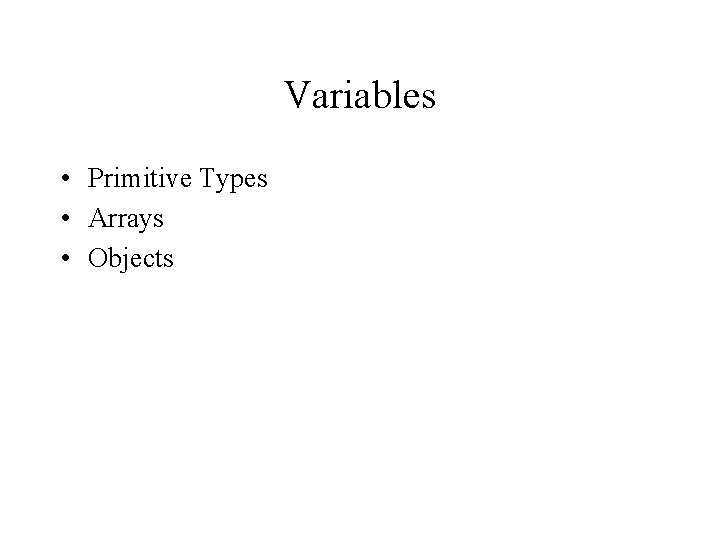Variables • Primitive Types • Arrays • Objects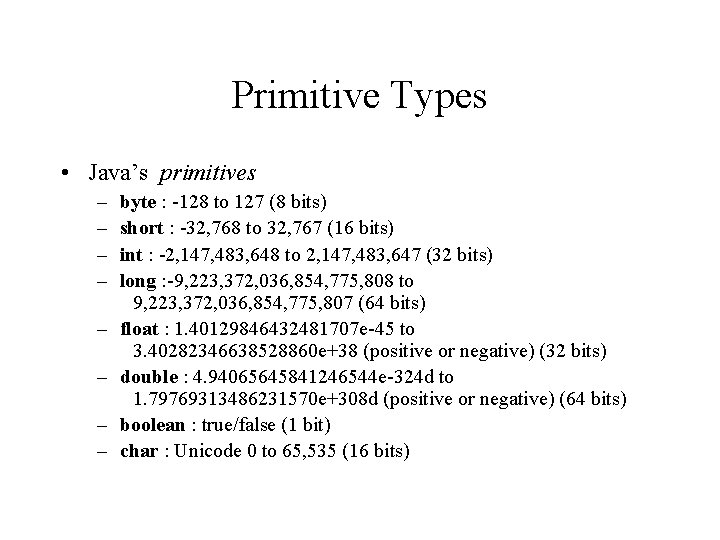Primitive Types • Java’s primitives – – – – byte : -128 to 127 (8 bits) short : -32, 768 to 32, 767 (16 bits) int : -2, 147, 483, 648 to 2, 147, 483, 647 (32 bits) long : -9, 223, 372, 036, 854, 775, 808 to 9, 223, 372, 036, 854, 775, 807 (64 bits) float : 1. 40129846432481707 e-45 to 3. 40282346638528860 e+38 (positive or negative) (32 bits) double : 4. 94065645841246544 e-324 d to 1. 79769313486231570 e+308 d (positive or negative) (64 bits) boolean : true/false (1 bit) char : Unicode 0 to 65, 535 (16 bits)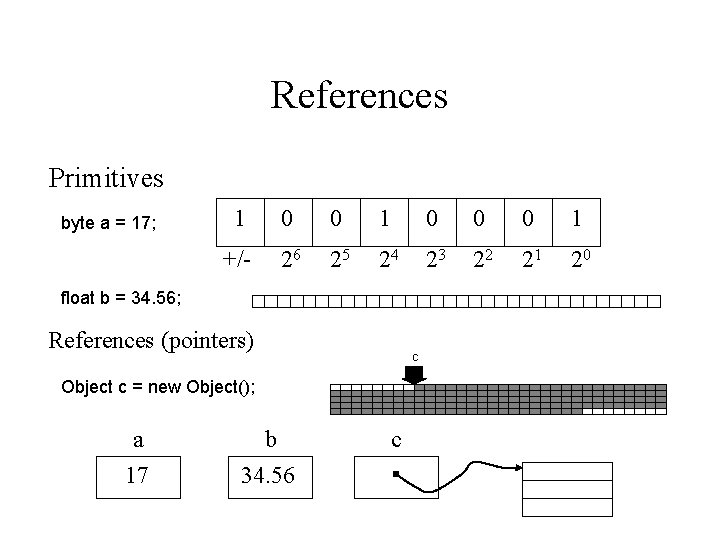References Primitives byte a = 17; 1 0 0 0 1 +/- 26 25 24 23 22 21 20 float b = 34. 56; References (pointers) c Object c = new Object(); a 17 b 34. 56 c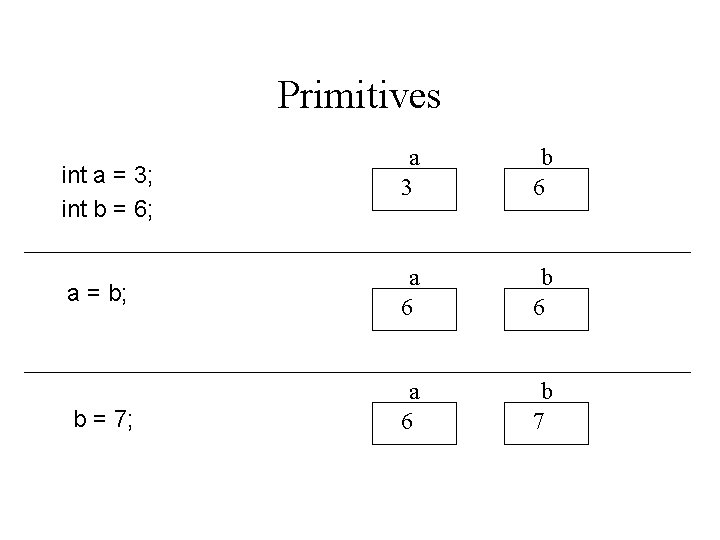Primitives int a = 3; int b = 6; a = b; b = 7; a 3 b 6 a 6 b 7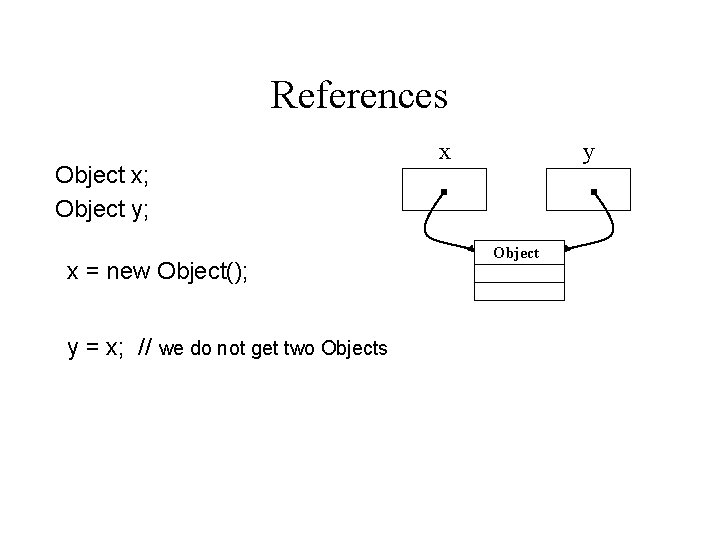References Object x; Object y; x = new Object(); y = x; // we do not get two Objects x y ObjectReferences Object x; Object y; x = new Object(); x y Object y = x. clone();Arrays • Array • A collection of a known number of variables of the same type. • In Java, it is a reference type – an object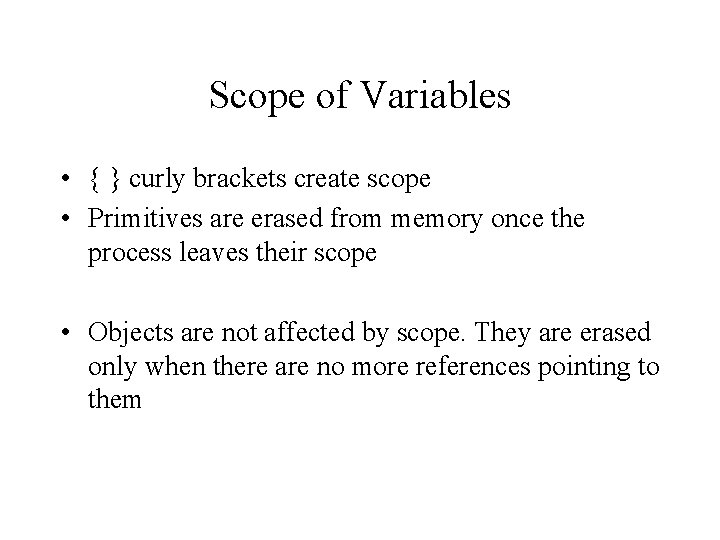Scope of Variables • { } curly brackets create scope • Primitives are erased from memory once the process leaves their scope • Objects are not affected by scope. They are erased only when there are no more references pointing to them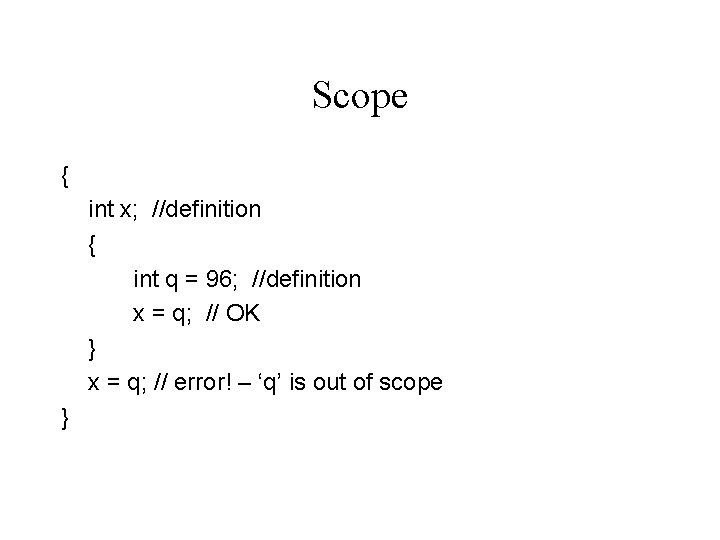Scope { int x; //definition { int q = 96; //definition x = q; // OK } x = q; // error! – ‘q’ is out of scope }Classes and Objects • Class is the definition for a new type • Instances of the class, objects, are created (instantiated) with the “new” operator. • Access to properties and methods of an object is by placing a ‘. ’ character between the object reference and the property or method name. an. Object. an. Int. Variable = 5; an. Object. a. Method(); an. Object. another. Object. a. Method(); • “object oriented”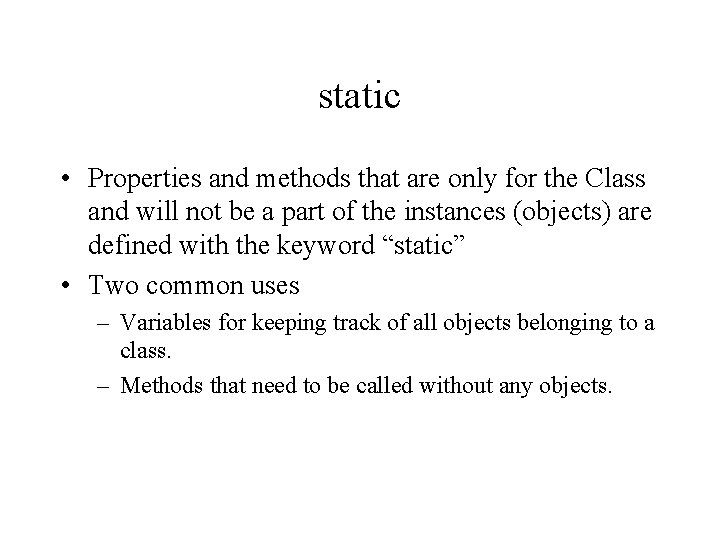static • Properties and methods that are only for the Class and will not be a part of the instances (objects) are defined with the keyword “static” • Two common uses – Variables for keeping track of all objects belonging to a class. – Methods that need to be called without any objects.Packages • “File system” to avoid naming conflicts between source codes from different developers. • In order to use a class in your code, you need to specify the package where it belongs by preceeding the class name with the package name. java. util. Array. List a = new java. util. Array. List(); • import statement provides a shorthand for the source code. import java. util. Array. List; . . . Array. List a = new Array. List(); • import java. lang. *; statement above is implied for every java program and need not be included.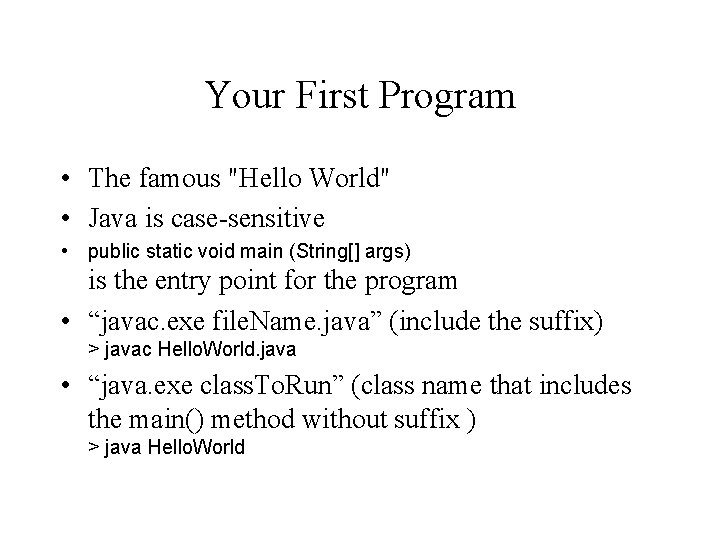Your First Program • The famous "Hello World" • Java is case-sensitive • public static void main (String[] args) is the entry point for the program • “javac. exe file. Name. java” (include the suffix) > javac Hello. World. java • “java. exe class. To. Run” (class name that includes the main() method without suffix ) > java Hello. World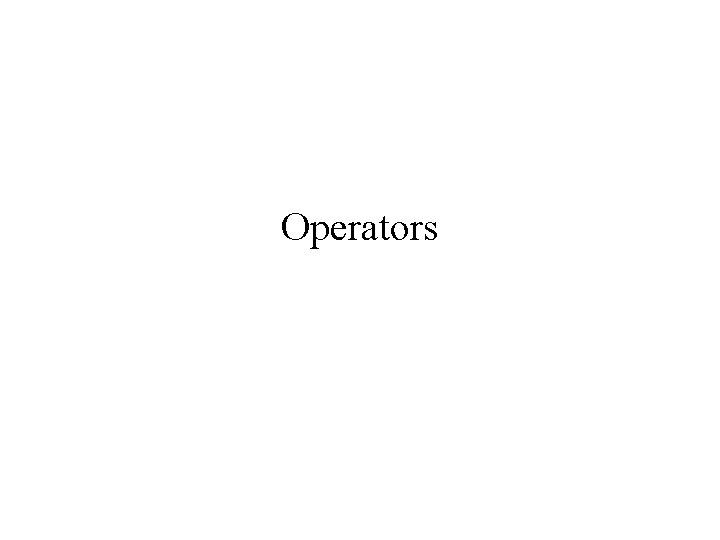Operators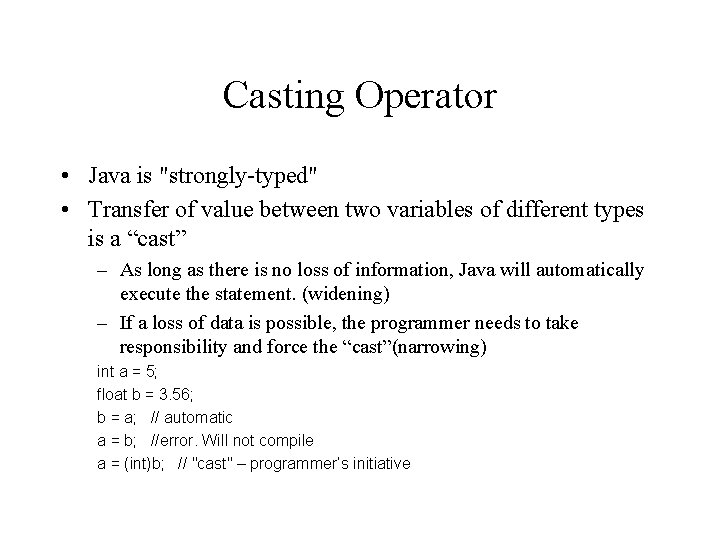Casting Operator • Java is "strongly-typed" • Transfer of value between two variables of different types is a “cast” – As long as there is no loss of information, Java will automatically execute the statement. (widening) – If a loss of data is possible, the programmer needs to take responsibility and force the “cast”(narrowing) int a = 5; float b = 3. 56; b = a; // automatic a = b; //error. Will not compile a = (int)b; // "cast" – programmer’s initiative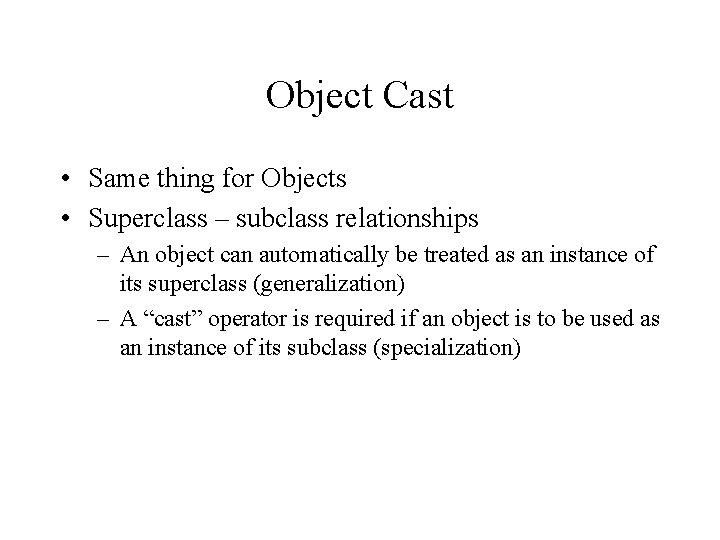Object Cast • Same thing for Objects • Superclass – subclass relationships – An object can automatically be treated as an instance of its superclass (generalization) – A “cast” operator is required if an object is to be used as an instance of its subclass (specialization)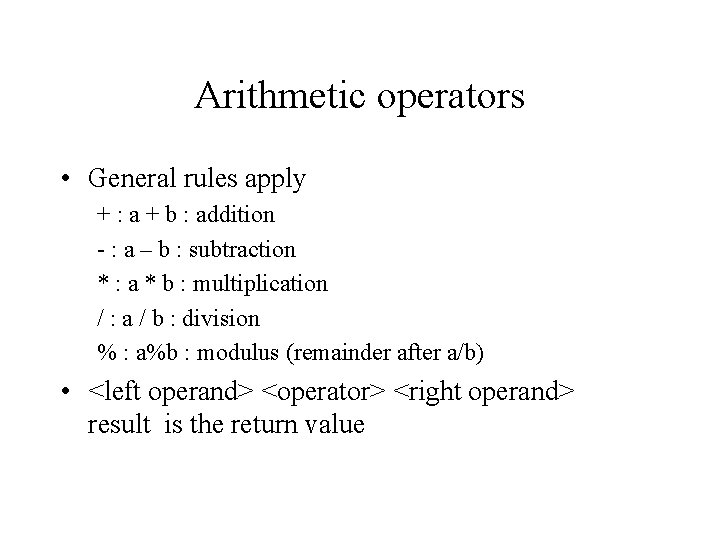Arithmetic operators • General rules apply + : a + b : addition - : a – b : subtraction * : a * b : multiplication / : a / b : division % : a%b : modulus (remainder after a/b) • <left operand> <operator> <right operand> result is the return value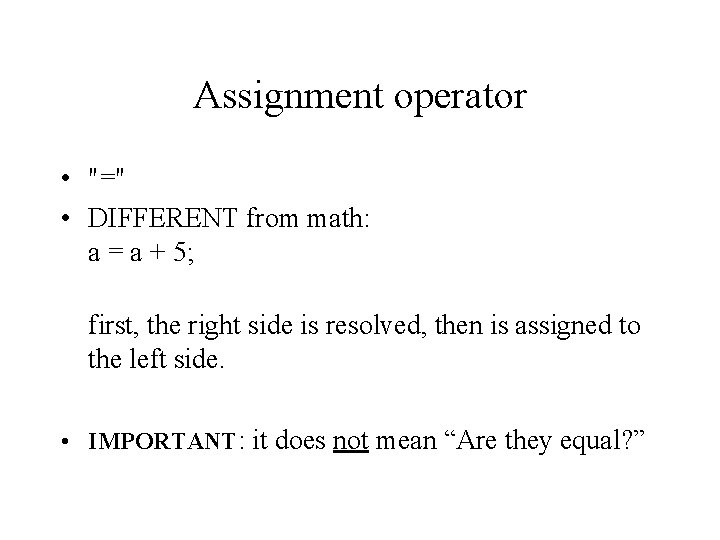Assignment operator • "=" • DIFFERENT from math: a = a + 5; first, the right side is resolved, then is assigned to the left side. • IMPORTANT: it does not mean “Are they equal? ”Shorthand for increment/decrement • ++a; a = a+1; equivalent (shorthand notation) – – ++counter; use the value after incrementing --counter ; use the value after decrementing counter++; use the value first, then increment counter--; use the value first, then decrementComparison operators • The operators below compare the right operand with the left operand return a boolean value (true or false) – – – > : a >b; >= : a>=b; < : a<b; <= : a<=b; == : a==b; != : a!=b; is a greater than b? is a greater than or equal to b? is a less than b? is a lass than or equal to b? ? is a equal to b? (note the double equals) is a not equal to b? • IMPORTANT: You should not compare objects with these operators.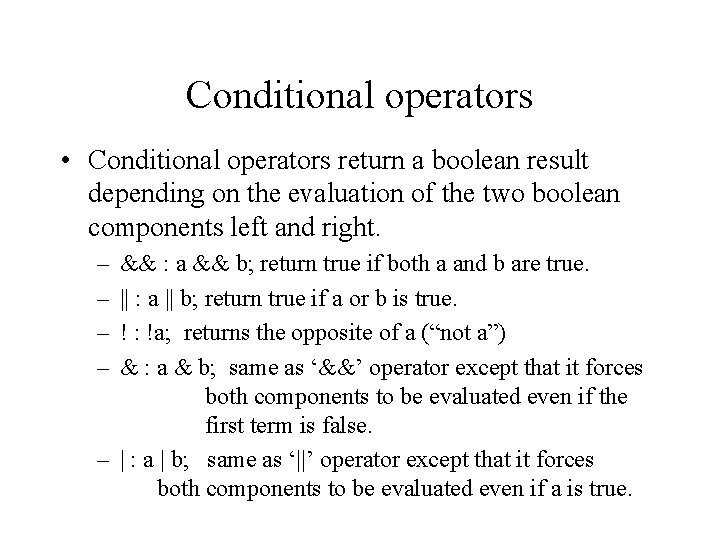Conditional operators • Conditional operators return a boolean result depending on the evaluation of the two boolean components left and right. – – && : a && b; return true if both a and b are true. || : a || b; return true if a or b is true. ! : !a; returns the opposite of a (“not a”) & : a & b; same as ‘&&’ operator except that it forces both components to be evaluated even if the first term is false. – | : a | b; same as ‘||’ operator except that it forces both components to be evaluated even if a is true.Promotion Rule • If an operator is used on two values which are of different types, the ‘smaller’ of the two types is promoted to the ‘larger’ type and then the evaluation is performed. For example, 3. 1415 * 7 • 3. 1415 is a float and 7 is an int. Since int is ‘smaller’, it is converted to a float 7. 0 and then the numbers are multiplied.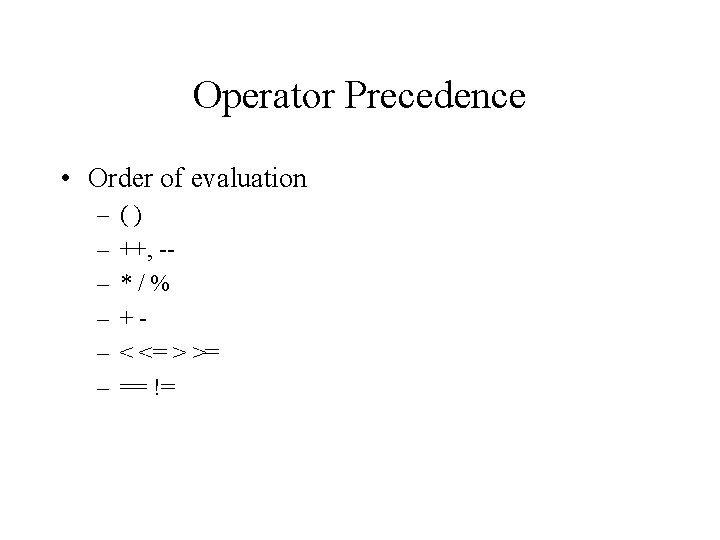Operator Precedence • Order of evaluation – – – () ++, -*/% +< <= > >= == !=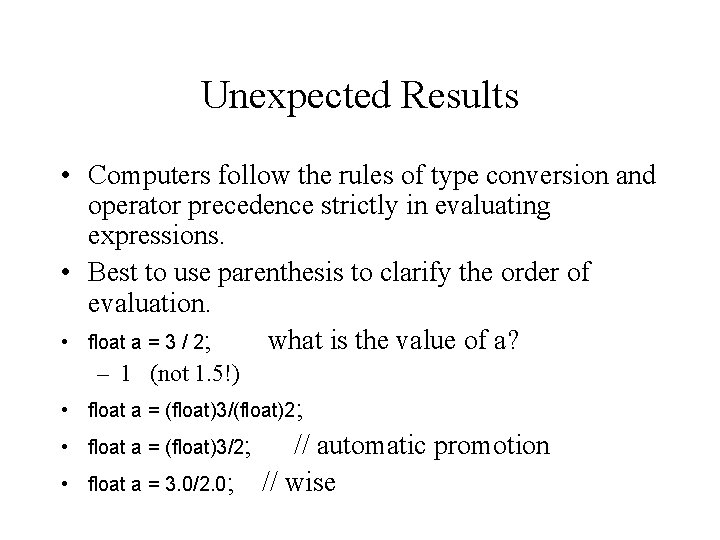Unexpected Results • Computers follow the rules of type conversion and operator precedence strictly in evaluating expressions. • Best to use parenthesis to clarify the order of evaluation. • float a = 3 / 2; what is the value of a? – 1 (not 1. 5!) • float a = (float)3/(float)2; • float a = (float)3/2; • // automatic promotion float a = 3. 0/2. 0; // wiseWrapper Classes • Recently called `Numbers Classes` • For each of the primitive data types, Java also provides a useful ‘wrapper’ Class in java. lang package – – – int : Integer long : Long float : Float double : Double char : Character boolean : Boolean • The wrapper classes provide a bridge between primitive data types and Objectsjava. lang. Math • The Math Class provides some basic mathematical functions. Examples: – – – static double sqrt(double a) static double random() static double sin() static double abs(double a) static double max(double a, double b) • As well as common Constants – Math. PI – Math. E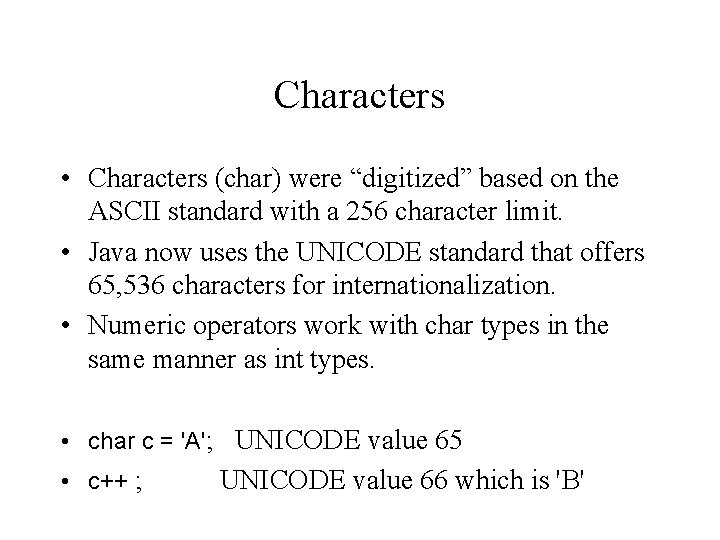Characters • Characters (char) were “digitized” based on the ASCII standard with a 256 character limit. • Java now uses the UNICODE standard that offers 65, 536 characters for internationalization. • Numeric operators work with char types in the same manner as int types. • char c = 'A'; UNICODE value 65 • c++ ; UNICODE value 66 which is 'B'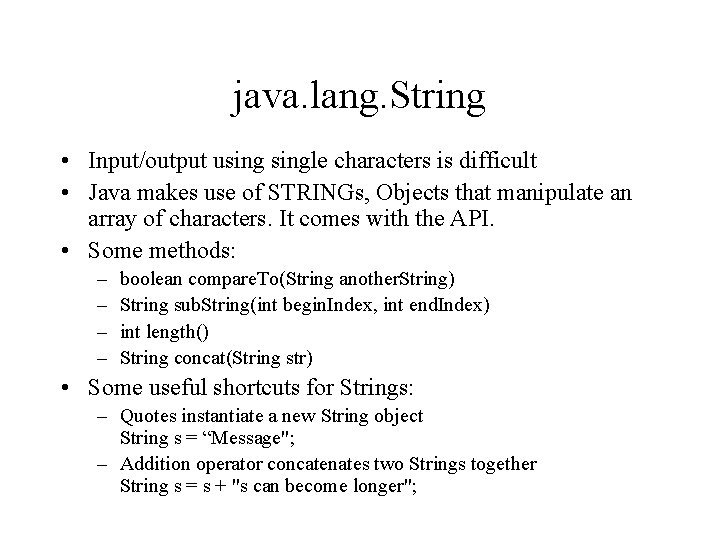java. lang. String • Input/output usingle characters is difficult • Java makes use of STRINGs, Objects that manipulate an array of characters. It comes with the API. • Some methods: – – boolean compare. To(String another. String) String sub. String(int begin. Index, int end. Index) int length() String concat(String str) • Some useful shortcuts for Strings: – Quotes instantiate a new String object String s = “Message"; – Addition operator concatenates two Strings together String s = s + "s can become longer";Assignment 1 • Write a “Circle” class – – • Two methods to calculate its area and circumference A third method to find out if a given point is inside the circle Write a “Circle. Application” class (separate. java file) – – Only a main() method Should create 5 circles, and a single point. It should output the following about each: • • Coordinates for its center Area Circumference If the point lies inside or outside the circle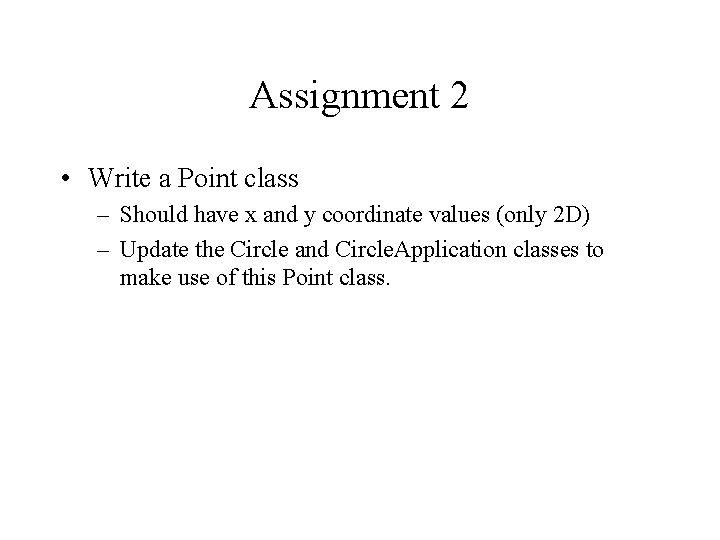Assignment 2 • Write a Point class – Should have x and y coordinate values (only 2 D) – Update the Circle and Circle. Application classes to make use of this Point class.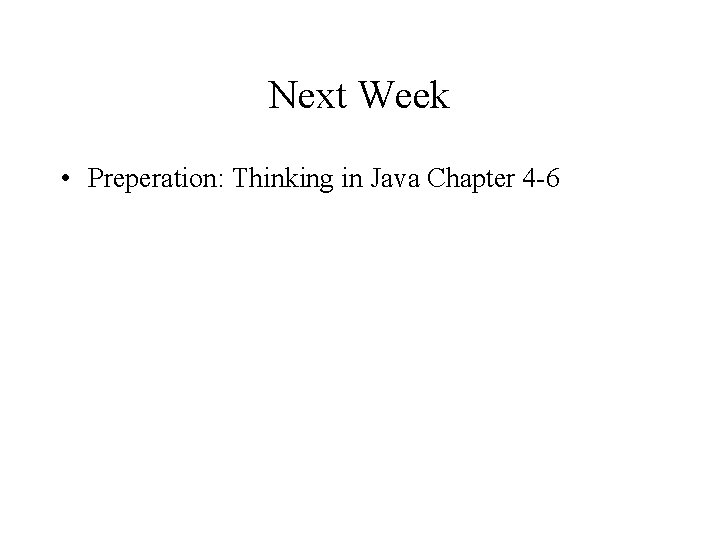Next Week • Preperation: Thinking in Java Chapter 4 -6